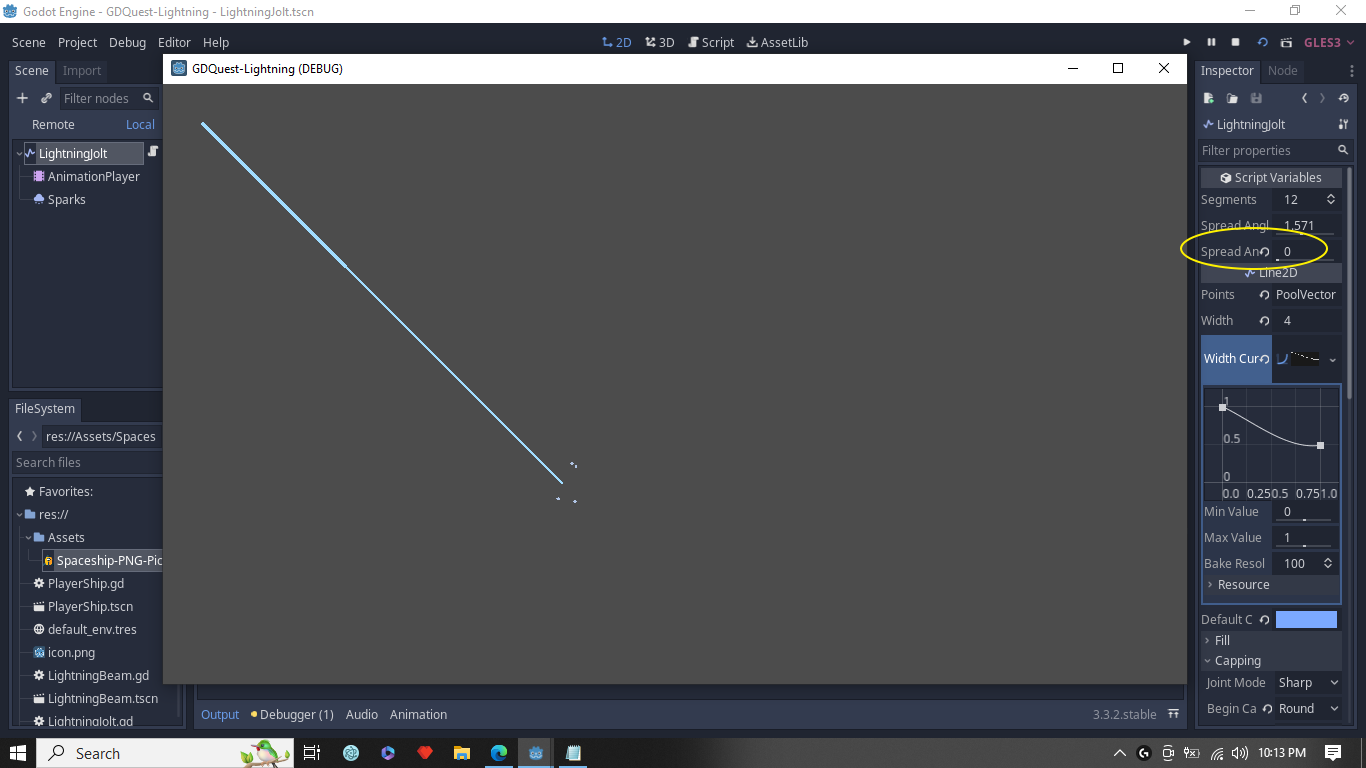c

# [Tip] How to control the shape of the lightning jolt.

carlo_montoya

[This is not a question but just want to share this with future learners]

Rewrite the following code:

`var _rotation := rand_range(-spread_angle / 2, spread_angle / 2)`

to

```var _angle := spread_angle * spread_angle_limit
```
`var _rotation := rand_range(-_angle, _angle)`

`export (float, 0.0, 0.5) var spread_angle_limit := 0.5`